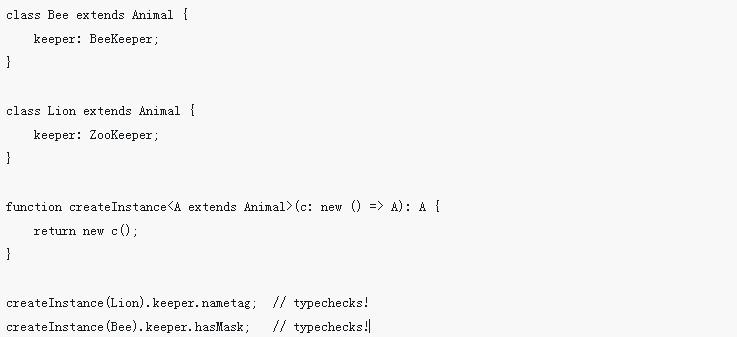# 泛型血雨探花 更新时间：2019-01-07 15:49:28

# 泛型之Hello World

```function identity(arg: number): number {
return arg;
}```

```function identity(arg: any): any {
return arg;
}```

```function identity<T>(arg: T): T {
return arg;
}```

`let output = identity<string>("myString");  // type of output will be 'string'`

`let output = identity("myString");  // type of output will be 'string'`

# 使用泛型变量

```function identity<T>(arg: T): T {
return arg;
}```

```function loggingIdentity<T>(arg: T): T {
console.log(arg.length);  // Error: T doesn't have .length
return arg;
}```

```function loggingIdentity<T>(arg: T[]): T[] {
console.log(arg.length);  // Array has a .length, so no more error
return arg;
}```

```function loggingIdentity<T>(arg: Array<T>): Array<T> {
console.log(arg.length);  // Array has a .length, so no more error
return arg;
}```

# 泛型类型

```function identity<T>(arg: T): T {
return arg;
}

let myIdentity: <T>(arg: T) => T = identity;```

```function identity<T>(arg: T): T {
return arg;
}

let myIdentity: <U>(arg: U) => U = identity;```

```function identity<T>(arg: T): T {
return arg;
}

let myIdentity: {<T>(arg: T): T} = identity;```

```interface GenericIdentityFn {    <T>(arg: T): T;
}

function identity<T>(arg: T): T {
return arg;
}

let myIdentity: GenericIdentityFn = identity;```

```interface GenericIdentityFn<T> {
(arg: T): T;
}

function identity<T>(arg: T): T {
return arg;
}

let myIdentity: GenericIdentityFn<number> = identity;```

# 泛型类

```class GenericNumber<T> {
zeroValue: T;
add: (x: T, y: T) => T;
}

let myGenericNumber = new GenericNumber<number>();
myGenericNumber.zeroValue = 0;
myGenericNumber.add = function(x, y) { return x + y; };```

`GenericNumber`类的使用是十分直观的，并且你可能已经注意到了，没有什么去限制它只能使用`number`类型。 也可以使用字符串或其它更复杂的类型。

```let stringNumeric = new GenericNumber<string>();
stringNumeric.zeroValue = "";
stringNumeric.add = function(x, y) { return x + y; };

alert(stringNumeric.add(stringNumeric.zeroValue, "test"));```

# 泛型约束

```function loggingIdentity<T>(arg: T): T {
console.log(arg.length);  // Error: T doesn't have .length
return arg;
}```

```interface Lengthwise {
length: number;
}

function loggingIdentity<T extends Lengthwise>(arg: T): T {
console.log(arg.length);  // Now we know it has a .length property, so no more error
return arg;
}```

`loggingIdentity(3);  // Error, number doesn't have a .length property`

`loggingIdentity({length: 10, value: 3});`

## 在泛型约束中使用类型参数

```function getProperty<T, K extends keyof T>(obj: T, key: K) {
return obj[key];
}

let x = { a: 1, b: 2, c: 3, d: 4 };

getProperty(x, "a"); // okay
getProperty(x, "m"); // error: Argument of type 'm' isn't assignable to 'a' | 'b' | 'c' | 'd'.```

## 在泛型里使用类类型

```function create<T>(c: {new(): T; }): T {
return new c();
}```

```class BeeKeeper {
hasMask: boolean;
}

class ZooKeeper {
nametag: string;
}

class Animal {
numLegs: number;
}

class Bee extends Animal {
keeper: BeeKeeper;
}

class Lion extends Animal {
keeper: ZooKeeper;
}

function createInstance<A extends Animal>(c: new () => A): A {
return new c();
}

createInstance(Lion).keeper.nametag;  // typechecks!
createInstance(Bee).keeper.hasMask;   // typechecks!```

php中文网
phpstudy

QQ交流群